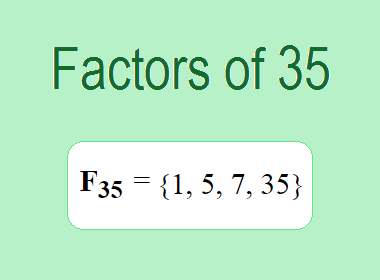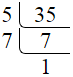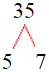# Factors of 35The factors of 35 are 1, 5, 7, and 35 i.e. F35 = {1, 5, 7, 35}. The factors of 35 are all the numbers that can divide 35 without leaving a remainder.

We can check if these numbers are factors of 35 by dividing 35 by each of them. If the result is a whole number, then the number is a factor of 35. Let's do this for each of the numbers listed above:

·        1 is a factor of 35 because 35 divided by 1 is 35.

·        5 is a factor of 35 because 35 divided by 5 is 7.

·        7 is a factor of 35 because 35 divided by 7 is 5.

·        35 is a factor of 35 because 35 divided by 35 is 1.

## How to Find Factors of 35?

1 and the number itself are the factors of every number. So, 1 and 35 are two factors of 35. To find the other factors of 35, we can start by dividing 35 by the numbers between 1 and 35. If we divide 35 by 2, we get a remainder of 1. Therefore, 2 is not a factor of 35. If we divide 35 by 3, we get a remainder of 2. Therefore, 3 is not a factor of 35.

Next, we can check if 4 is a factor of 35. If we divide 35 by 4, we get a remainder of 3. Therefore, 4 is not a factor of 35. If we divide 35 by 5, we get a remainder of 0. Therefore, 5 is a factor of 35. We can continue this process for all the possible factors of 35.

Through this process, we can find that the factors of 35 are 1, 5, 7, and 35. These are the only numbers that can divide 35 without leaving a remainder.

********************

********************

## Properties of the Factors of 35

The factors of 35 have some interesting properties. One of the properties is that the sum of the factors of 35 is equal to 48. We can see this by adding all the factors of 35 together:

1 + 5 + 7 + 35 = 48

Another property of the factors of 35 is that they are all odd numbers. This is because 35 is an odd number, and any even number cannot divide an odd number without leaving a remainder.

Another property of the factors of 35 is that the prime factors of 35 are 5 and 7 only.

## Applications of the Factors of 35

The factors of 35 have several applications in mathematics. One of the applications is in finding the highest common factor (HCF) of two or more numbers. The HCF is the largest factor that two or more numbers have in common. For example, to find the HCF of 35 and 28, we need to find the factors of both numbers and identify the largest factor they have in common. The factors of 35 are 1, 5, 7, and 35. The factors of 28 are 1, 2, 4, 7, 14, and 28. The largest factor that they have in common is 7. Therefore, the HCF of 35 and 28 is 7.

Another application of the factors of 35 is in prime factorization. Prime factorization is the process of expressing a number as the product of its prime factors. The prime factors of 35 are 5 and 7, since these are the only prime numbers that can divide 35 without leaving a remainder. Therefore, we can express 35 as:

35 = 5 × 7

We can do prime factorization by division and factor tree method also. Here is the prime factorization of 35 by division method,35 = 5 × 7

Here is the prime factorization of 35 by the factor tree method,35 = 5 × 7

## Conclusion

The factors of 35 are the numbers that can divide 35 without leaving a remainder. The factors of 35 are 1, 5, 7, and 35. The factors of 35 have some interesting properties, such as being odd numbers and having a sum of 48. The factors of 35 have several applications in mathematics, such as finding the highest common factor and prime factorization.